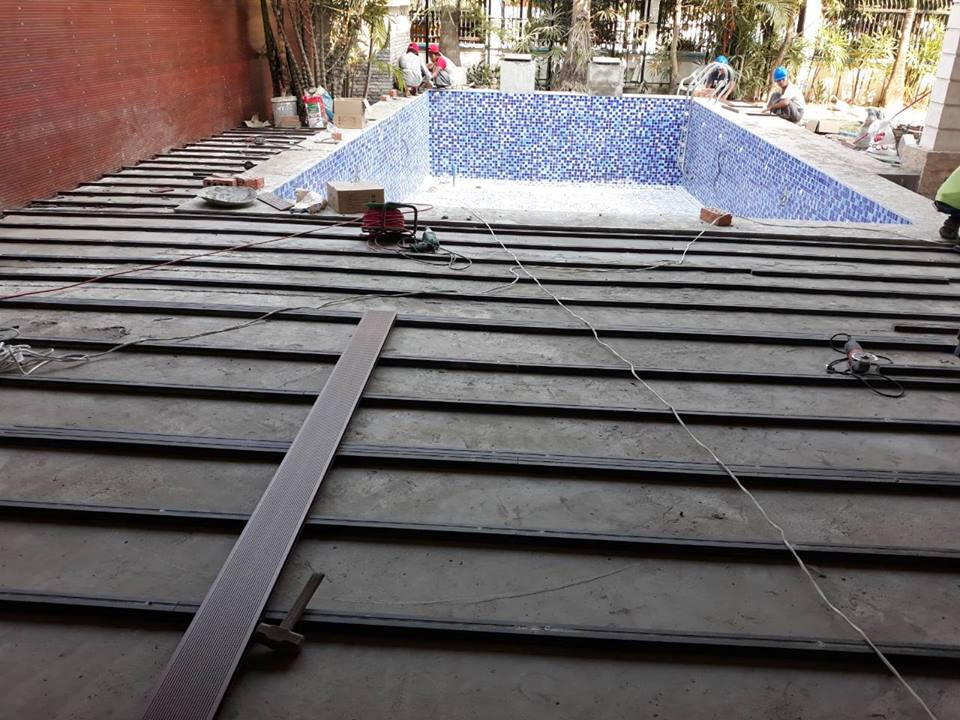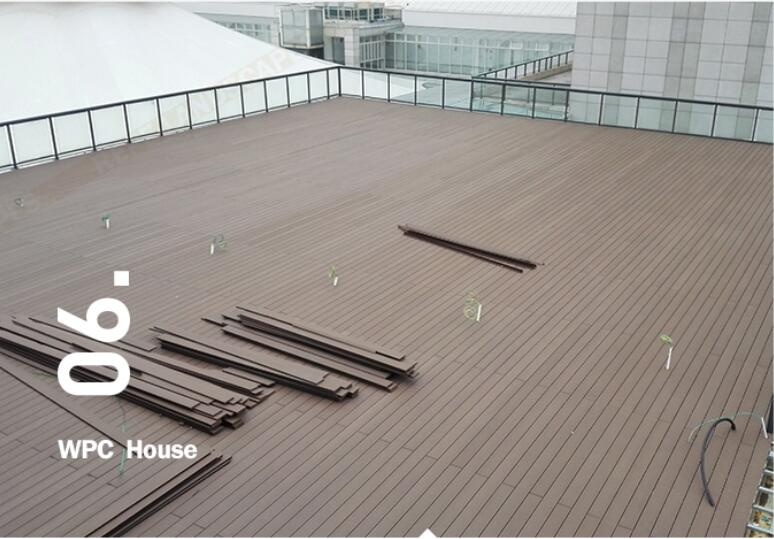# wpc decking calculator | how to calculate how much floor this place needs

Many customers just don’t know how to calculate how much floor this place needs. We often encounter such customers, they know the area but do not know how many floors need to be customized.

Let’s take an example. Mr LUKE Has a Project of WPC decking in Malta.

The ground size : length—–50meters,   width—–2m,  total will be 100 sqms.

How much material does it take? Let me calculate for him. if he order 140X25mm from us.length is 2.2m/PC

One sqm need 7.1428m decking , So 100sqms need 714m, if the length just is 2m Width,  total need 357 PCS.

But now the ordered length is 2.2m,  we need cut 0.2m from every PCS, we said 100sqm need 714m, So if don’t have any waste, it will be 325PCS. but because of the 0.2m waste ,we still need 357PCS.

The gap between two joists are 300mm. We need  150 PCS WPC Joist with the length of 2m.

CLIPS+ Screws need 2400 SETS. ( One sqm decking need about 24 Sets )The most common question we’re asked is “How many deckings will I need?”.” How many PCS of Clips does one sqm WPC decking need ？“”

Our calculator below should be able to give you an idea of how much decking boards you will need, and. Of course if you wish to speak to one of our sales for a much more accurate quote then please Contact with us by Admin@hanmingwpc.com

1:Start with the area you want, in square metres. To do this, simply measure the area of the meter and multiply the width by the depth. If the area you are doing is not a simple rectangle, you need to divide it into several rectangles and calculate each area separately.Step 2：

The number of WPC deckings  required depends on the  length of the borads(2.2n, 2.9m,3m,4m,4.5m，5.4m)